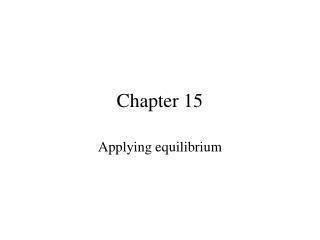DownloadDownload PresentationChapter 15

# Chapter 15

Download Presentation## Chapter 15

- - - - - - - - - - - - - - - - - - - - - - - - - - - E N D - - - - - - - - - - - - - - - - - - - - - - - - - - -
##### Presentation Transcript

1. Chapter 15 Applying equilibrium

2. Titration Curves

3. Strong acid with strong Base • Equivalence at pH 7 7 pH mL of Base added

4. Weak acid with strong Base • Equivalence at pH >7 >7 pH mL of Base added

5. Strong base with strong acid • Equivalence at pH 7 7 pH mL of Base added

6. Weak base with strong acid • Equivalence at pH <7 <7 pH mL of Base added

7. Summary • Strong acid and base just stoichiometry. • Determine Ka, use for 0 mL base • Weak acid before equivalence point • Stoichiometry first • Then Henderson-Hasselbach • Weak acid at equivalence point Kb • Weak base after equivalence - leftover strong base.

8. Summary • Determine Ka, use for 0 mL acid. • Weak base before equivalence point. • Stoichiometry first • Then Henderson-Hasselbach • Weak base at equivalence point Ka. • Weak base after equivalence - leftover strong acid.

9. Indicators • Weak acids that change color when they become bases. • weak acid written HIn • Weak base • HIn H+ + In- clear red • Equilibrium is controlled by pH • End point - when the indicator changes color.

10. Indicators • Since it is an equilibrium the color change is gradual. • It is noticeable when the ratio of [In-]/[HI] or [HI]/[In-] is 1/10 • Since the Indicator is a weak acid, it has a Ka. • pH=pKa +log([In-]/[HI]) = pKa +log(1/10) • pH=pKa - 1 on the way up

11. Indicators • pH=pKa + log([HI]/[In-]) = pKa + log(10) • pH=pKa +1 on the way down • Choose the indicator with a pKa 1 less than the pH at equivalence point if you are titrating with base. • Choose the indicator with a pKa 1 greater than the pH at equivalence point if you are titrating with acid.